# Mesh Parameterization¶

Important: Please read the installation page for details about how to install the toolboxes. $\newcommand{\dotp}{\langle #1, #2 \rangle}$ $\newcommand{\enscond}{\lbrace #1, #2 \rbrace}$ $\newcommand{\pd}{ \frac{ \partial #1}{\partial #2} }$ $\newcommand{\umin}{\underset{#1}{\min}\;}$ $\newcommand{\umax}{\underset{#1}{\max}\;}$ $\newcommand{\umin}{\underset{#1}{\min}\;}$ $\newcommand{\uargmin}{\underset{#1}{argmin}\;}$ $\newcommand{\norm}{\|#1\|}$ $\newcommand{\abs}{\left|#1\right|}$ $\newcommand{\choice}{ \left\{ \begin{array}{l} #1 \end{array} \right. }$ $\newcommand{\pa}{\left(#1\right)}$ $\newcommand{\diag}{{diag}\left( #1 \right)}$ $\newcommand{\qandq}{\quad\text{and}\quad}$ $\newcommand{\qwhereq}{\quad\text{where}\quad}$ $\newcommand{\qifq}{ \quad \text{if} \quad }$ $\newcommand{\qarrq}{ \quad \Longrightarrow \quad }$ $\newcommand{\ZZ}{\mathbb{Z}}$ $\newcommand{\CC}{\mathbb{C}}$ $\newcommand{\RR}{\mathbb{R}}$ $\newcommand{\EE}{\mathbb{E}}$ $\newcommand{\Zz}{\mathcal{Z}}$ $\newcommand{\Ww}{\mathcal{W}}$ $\newcommand{\Vv}{\mathcal{V}}$ $\newcommand{\Nn}{\mathcal{N}}$ $\newcommand{\NN}{\mathcal{N}}$ $\newcommand{\Hh}{\mathcal{H}}$ $\newcommand{\Bb}{\mathcal{B}}$ $\newcommand{\Ee}{\mathcal{E}}$ $\newcommand{\Cc}{\mathcal{C}}$ $\newcommand{\Gg}{\mathcal{G}}$ $\newcommand{\Ss}{\mathcal{S}}$ $\newcommand{\Pp}{\mathcal{P}}$ $\newcommand{\Ff}{\mathcal{F}}$ $\newcommand{\Xx}{\mathcal{X}}$ $\newcommand{\Mm}{\mathcal{M}}$ $\newcommand{\Ii}{\mathcal{I}}$ $\newcommand{\Dd}{\mathcal{D}}$ $\newcommand{\Ll}{\mathcal{L}}$ $\newcommand{\Tt}{\mathcal{T}}$ $\newcommand{\si}{\sigma}$ $\newcommand{\al}{\alpha}$ $\newcommand{\la}{\lambda}$ $\newcommand{\ga}{\gamma}$ $\newcommand{\Ga}{\Gamma}$ $\newcommand{\La}{\Lambda}$ $\newcommand{\si}{\sigma}$ $\newcommand{\Si}{\Sigma}$ $\newcommand{\be}{\beta}$ $\newcommand{\de}{\delta}$ $\newcommand{\De}{\Delta}$ $\newcommand{\phi}{\varphi}$ $\newcommand{\th}{\theta}$ $\newcommand{\om}{\omega}$ $\newcommand{\Om}{\Omega}$

This tour explores 2-D parameterization of 3D surfaces using linear methods.

A review paper for mesh parameterization can be found in:

M.S. Floater and K. Hormann, Surface Parameterization: a Tutorial and Survey in Advances in multiresolution for geometric modelling, p. 157-186, 2005.

K. Hormann, K. Polthier and A. Sheffer Mesh parameterization: theory and practice, Siggraph Asia Course Notes

In :
using PyPlot
using NtToolBox
using Interpolations


## Conformal Laplacian¶

The conformal Laplacian uses the cotan weights to obtain an accurate discretization of the Laplace Beltrami Laplacian.

They where first introduces as a linear finite element approximation of the Laplace-Beltrami operator in:

U. Pinkall and K. Polthier, Computing discrete minimal surfaces and their conjugates Experimental Mathematics, 2(1):15-36, 1993.

First load a mesh. The faces are stored in a matrix $F = (f_j)_{j=1}^m \in \RR^{3 \times m}$ of $m$ faces $f_j \in \{1,\ldots,n\}^3$. The position of the vertices are stored in a matrix $X = (x_i)_{i=1}^n \in \RR^{3 \times n}$ of $n$ triplets of points $x_k \in \RR^3$

In :
X, F = read_mesh("NtToolbox/src/data/nefertiti.off")
n = size(X,2);


In order to perform mesh parameterization, it is important that this mesh has the topology of a disk, i.e. it should have a single B.

First we compute the boundary $B = (i_1,\ldots,i_p)$ of the mesh. By definition, for the edges $(i_k,i_{k+1})$, there is a single adjacent face $(i_k,i_{k+1},\ell)$.

In :
B = compute_boundary(F);


Length of the boundary.

In :
p = length(B);


Display the boundary.

In :
figure(figsize=(10,10))
#plot mesh
plot_mesh(X, F, el=80,az=-100, lwdt=.6, dist=6)

#plot boundary
for i in 1:length(B)
plot(X[1,B], X[2,B], X[3,B], color="red", lw=3)
end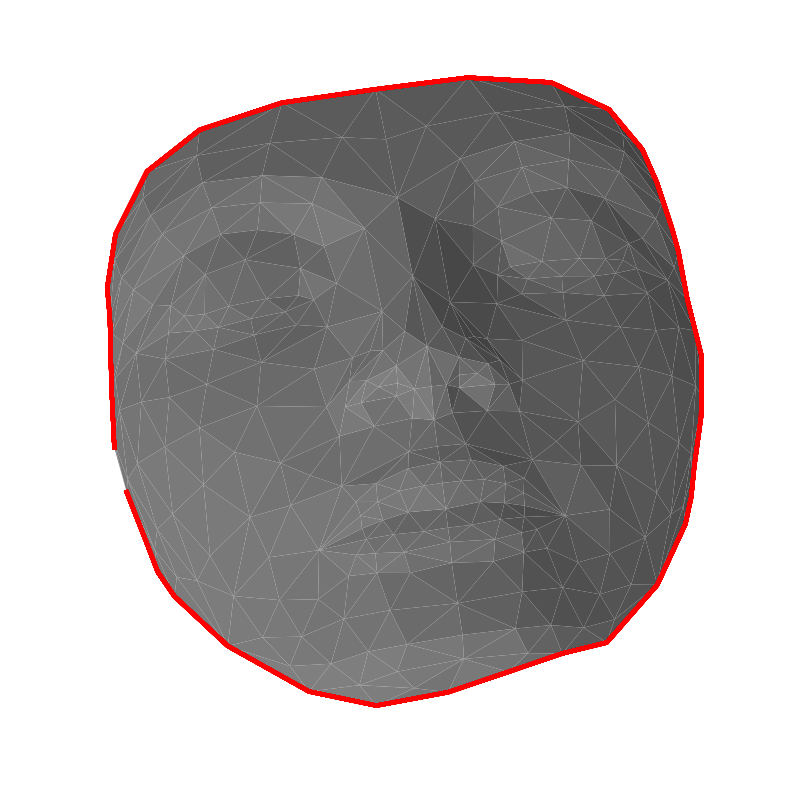The conformal Laplacian weight matrix $W \in \RR^{n \times n}$ is defined as $$W_{i,j} = \choice{ \text{cotan}(\al_{i,j}) + \text{cotan}(\be_{i,j}) \qifq i \sim j \\ \quad 0 \quad \text{otherwise}. }$$ Here, $i \times j$ means that there exists two faces $(i,j,k)$ and $(i,j,\ell)$ in the mesh (note that for B faces, one has $k=\ell$).

The angles are the angles centered as $k$ and $\ell$, i.e. $$\al_{i,j} = \widehat{x_i x_k x_j } \qandq \be_{i,j} = \widehat{x_i x_\ell x_j }.$$

Compute the conformal 'cotan' weights. Note that each angle $\alpha$ in the mesh contributes with $1/\text{tan}(\alpha)$ to the weight of the opposite edge. We compute $\alpha$ as $$\alpha = \text{acos}\pa{ \frac{\dotp{u}{v}}{\norm{u}\norm{v}} }$$ where $u \in \RR^3, v \in \RR^3$ are the edges of the adjacent vertices that defines $\al$.

In :
W = spzeros(n,n)

for i in 1:3
i2 = i%3+1
i3 = (i+1)%3+1
u = X[:,F[i2,:]] - X[:,F[i,:]]
v = X[:,F[i3,:]] - X[:,F[i,:]]
# normalize the vectors
u = u ./ repeat(sqrt(sum(u.^2,1)), outer=(3,1))
v = v ./ repeat(sqrt(sum(v.^2,1)), outer=(3,1))
# compute angles
alpha = 1./tan(acos(sum(u.*v, 1)))
alpha = vec(max(alpha, 1e-2)') #avoid degeneracy
W = W + sparse(F[i2,:],F[i3,:], alpha, n,n)
W = W + sparse(F[i3,:],F[i2,:], alpha, n,n)
end;


Compute the symmetric Laplacian matrix $L = D-W$ where $D = \mathrm{Diag}_i\pa{\sum_j W_{i,j}}$

In :
d = sum(W,1)
D = spdiagm(vec(d), 0,n,n)
L = D - W;


## Fixed Boundary Harmonic Parameterization¶

The problem of mesh parameterization corresponds to finding 2-D locations $(y_i = (y_i^1,y_i^2) \in \RR^2$ for each original vertex, where $Y = (y_i)_{i=1}^n \in \RR^{2 \times n}$ denotes the flattened positions.

The goal is for this parameterization to be valid, i.e. the 2-D mesh obtained by replacing $X$ by $Y$ but keeping the same face connectivity $F$ should not contained flipped faces (all face should have the same orientation in the plane).

We consider here a linear methods, that finds the parameterization, that impose that the coordinates are harmonic inside the domain, and have fixed position on the boundary (Dirichlet conditions) $$\forall s=1,2, \quad \forall i \notin B, \quad (L y^s)_i = 0, \qandq \forall j \in B, \quad y^s_j = z_j^s.$$

In order for this method to define a valid parameterization, it is necessary that the fixed position $z_j = (z^1_j,z^2_j) \in \RR^2$ are consecutive points along a convex polygon.

Compute the fixed positions $Z=(z_j)_j$ for the vertices on $B$. Here we use a circle.

In :
p = length(B)
t = collect(linspace(0,2*pi,p+1))
deleteat!(t,p+1)
Z = [cos(t)'; sin(t)'];


Computing the parameterization requires to solve two independent linear system $$\forall s=1,2, \quad L_1 y^s = r^s$$ where $L_1$ is a modified Laplacian, the is obtained from $L$ by $$\choice{ \forall i \notin B, \quad (L_0)_{i,j} = L_{i,j} \\ \forall i \in B, \quad (L_0)_{i,i}=1, \\ \forall i \in B, \forall j \neq i, \quad (L_0)_{i,i}=0, }$$ i.e. replacing each row indexed by $B$ by a 1 on the diagonal.

In :
L1 = copy(L)
L1[B,:] = 0
for i in 1:length(B)
L1[B[i], B[i]] = 1
end;


Set up the right hand size $R$ with the fixed position.

In :
R = zeros(2,n)
R[:,B] = Z;


Solve the two linear systems.

In :
Y = zeros(2,n)
Y = (L1 \ R')';


# Display the parameterization.¶

In :
figure(figsize=(10,10))
plot_mesh([Y;zeros(1,n)],F, lwdt = 1., c="lightgrey");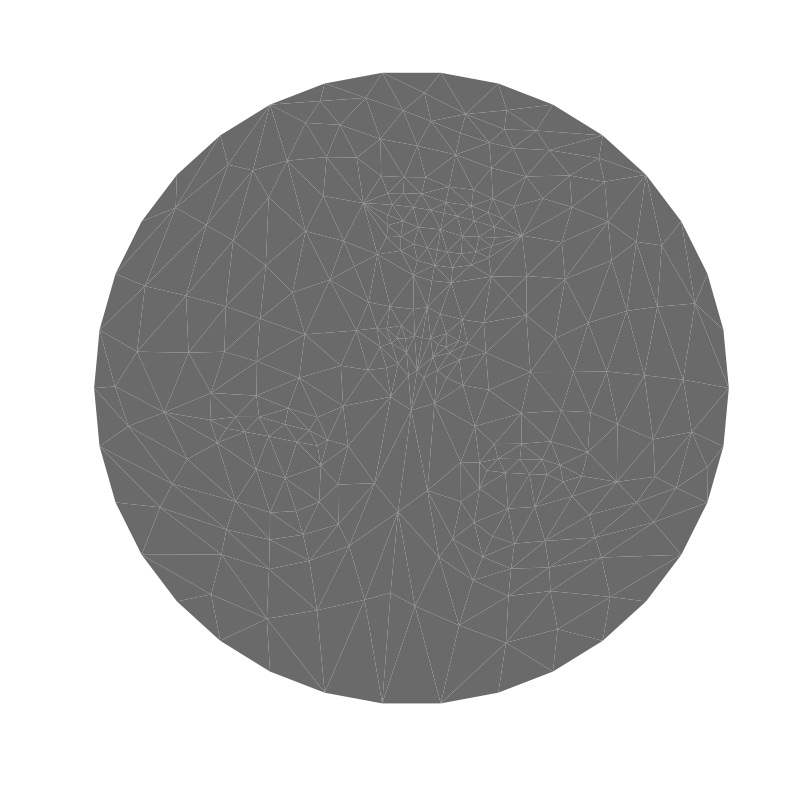## Mesh Parameterization on a Square¶

One can perform a fixed B parameterization on a square. This is useful to compute a geometry image (a color image storring the position of the vertices).

Exercise 1

Compute the fixed positions $Z$ of the points indexed by $B$ that are along a square. Warning: $p$ is not divisible by 4.

In :
include("NtSolutions/meshdeform_1_parameterization/exo1.jl");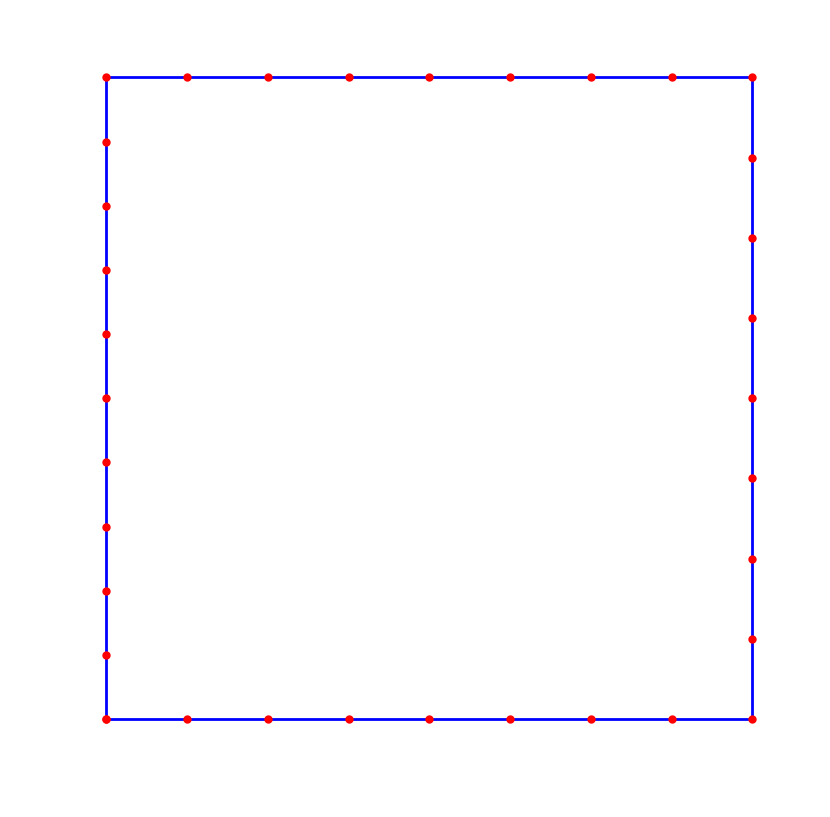In :
## Insert your code here.


Exercise 2

Compute the parameterization $Y$ on a square.

In :
include("NtSolutions/meshdeform_1_parameterization/exo2.jl");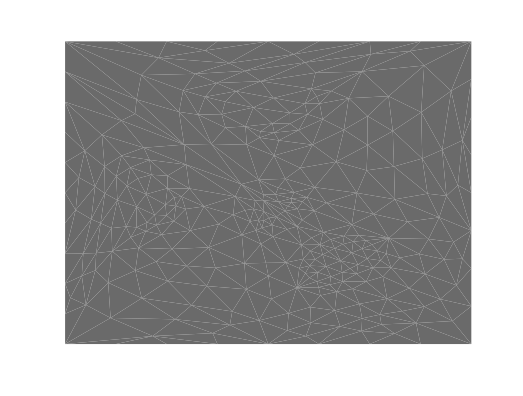Exercise 3

Shift the $B$ positions so that the eyes of the model are approximately horizontal.

In :
include("NtSolutions/meshdeform_1_parameterization/exo3.jl");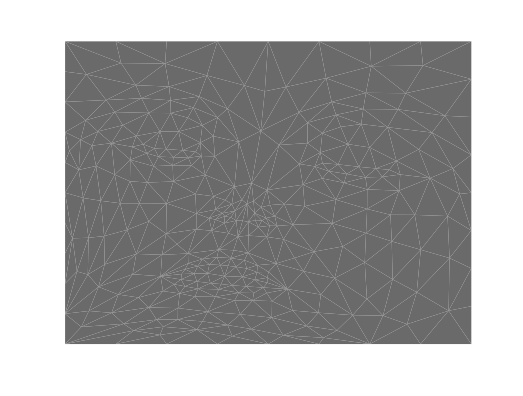In :
## Insert your code here.


## Re-align the Texture¶

To map correctly a real image on the surface, the texture needs to be aligned. We use here a simple affine mapping to map the eye and mouth of the image on the corresponding location on the surface.

Load a texture image $T$.

In :
n1 = 256
T = T[n1:-1:1,:];

UndefVarError: Images not defined

in load_image(::String, ::Int64, ::Int64, ::Int64, ::Int64) at /Users/quentin/.julia/v0.5/NtToolBox/src/signal.jl:33
in load_image(::String, ::Int64) at /Users/quentin/.julia/v0.5/NtToolBox/src/signal.jl:12
In :
C = rand(1000,3)
C = C./repeat(sum(C,2),outer=(1,3))
C=C[:,[3,2,1]]
C= sortcols(C)
lambd=C';


Display the texture on the mesh, using the parametrization of the mesh as texture coordinates.

In :
fig = figure(figsize = (10,10))
ax = subplot(111, projection="3d")

x = collect(linspace(0, 1, size(T,1)))
#itp = interpolate(T', BSpline(Cubic(Line())), OnGrid())
itp = interpolate((x,x), T', Gridded(Linear()))

for i in 1:size(F,2)
px = Y[1,F[:,i]]
py = Y[2,F[:,i]]

points_x = lambd'*px
points_y = lambd'*py
points = [points_x ; points_y]

col = zeros(size(points_x))
for ind in 1:size(points_x,1)
col[ind] = itp[points_x[ind], points_y[ind]]
end
col = rescale(col)
col = repeat(col,outer=(1,3))

Px = X[1,F[:,i]]
Py = X[2,F[:,i]]
Pz = X[3,F[:,i]]

Points_x = lambd'*Px
Points_y = lambd'*Py
Points_z = lambd'*Pz

scatter3D(Points_x,Points_y,Points_z,s=5,c=col,lw=0,alpha=1)
ax[:view_init](80, -100)
axis("off")
ax[:dist]=6

end

UndefVarError: T not defined


Position $(u_1,u_2,u_3)$ of the eyes and the mouth in the texture.

In :
u1 = [247,266]*n1/512.
u2 = [247,328]*n1/512.
u3 = [161,301]*n1/512.;


Display.

In :
figure(figsize=(10,10))
imageplot(T)
plot([u1,u2,u3,u1], [u1,u2,u3,u1], ".-b", ms=10, linewidth=2)
gca()[:invert_yaxis]()

UndefVarError: T not defined


Positions $(v_1,v_2,v_3)$ of the eyes and the mouth on the parameteric domain

In :
v1 = [310,125]*n1/512.
v2 = [315,350]*n1/512.
v3 = [105,232]*n1/512.;


Display.

In :
figure(figsize=(10,10))
axis("off")

Y_big = Y*(n1-1) + 1
beg = findn(W)
last = findn(W)

for i in 1:length(beg)
plot([Y_big[1, beg[i]], Y_big[1,last[i]]],[Y_big[2,beg[i]], Y_big[2,last[i]]],c="blue",lw=2)
end

plot([v1,v2,v3,v1], [v1,v2,v3,v1], ".-r", ms=10, linewidth=3);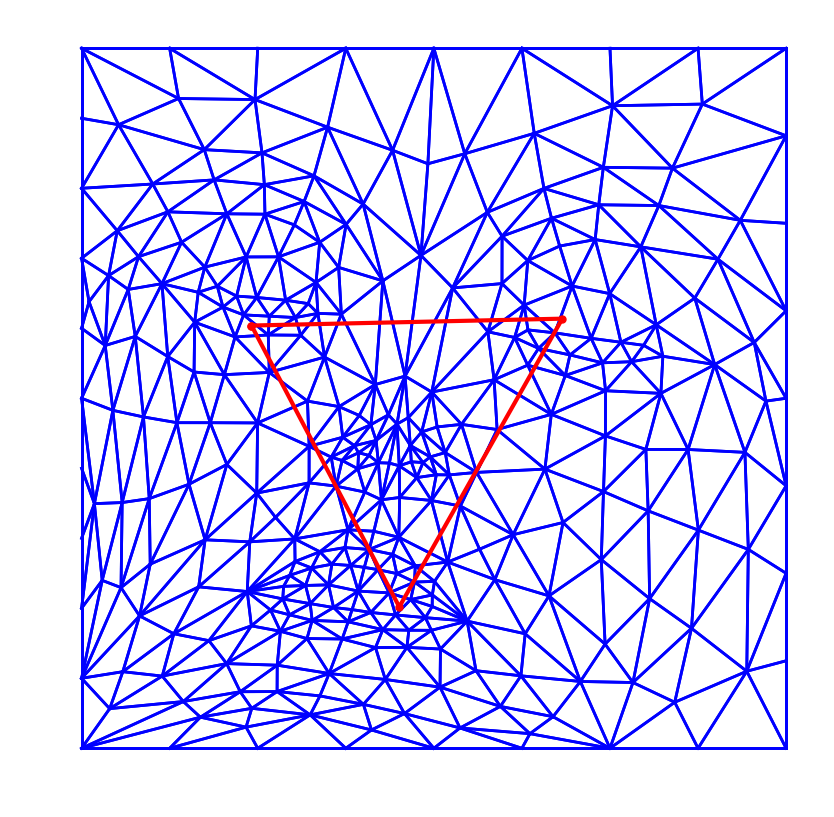Apply a similitude to the image so that the mouth and the eye have the correct position in parametric domain.

In :
T1 = perform_image_similitude(T,"affine",(u1-1)/n1,(v1-1)/n1,(u2-1)/n1,(v2-1)/n1,(u3-1)/n1,(v3-1)/n1);

UndefVarError: perform_image_similitude not defined


Display the mesh with the image overlaid.

In :
figure(figsize=(10,10))
axis("off")

Y_big = Y*(n1-1) + 1
beg = findn(W)
last = findn(W)

for i in 1:length(beg)
plot([Y_big[1, beg[i]], Y_big[1,last[i]]],[Y_big[2,beg[i]], Y_big[2,last[i]]],c="blue",lw=2)
end

plot([v1,v2,v3,v1], [v1,v2,v3,v1], ".-r", ms=10, linewidth=3)
imageplot(T1)
gca()[:invert_yaxis]()

UndefVarError: T1 not defined


Display the texture mapped mesh.

In :
fig = figure(figsize = (10,10))
ax = subplot(111, projection="3d")

x = collect(linspace(0, 1, size(T,1)))
itp = interpolate((x,x), T1', Gridded(Linear()))

for i in 1:size(F,2)
px = Y[1,F[:,i]]
py = Y[2,F[:,i]]

points_x = lambd'*px
points_y = lambd'*py
points = [points_x ; points_y]

col = zeros(size(points_x))
for ind in 1:size(points_x,1)
col[ind] = itp[points_x[ind], points_y[ind]]
end
col = rescale(col)
col = repeat(col,outer=(1,3))

Px = X[1,F[:,i]]
Py = X[2,F[:,i]]
Pz = X[3,F[:,i]]

Points_x = lambd'*Px
Points_y = lambd'*Py
Points_z = lambd'*Pz

scatter3D(Points_x,Points_y,Points_z,s=6,c=col,lw=0,alpha=1)
ax[:view_init](80, -100)
axis("off")
ax[:dist]=6

end

UndefVarError: T not defined